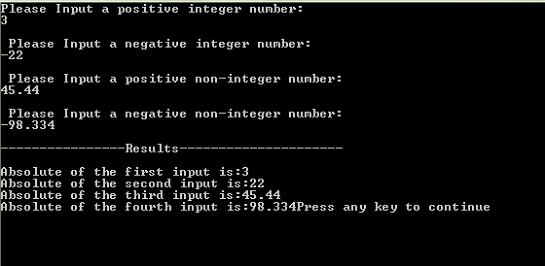# Function Templates in C++

In this tutorial you will learn:
1. What are templates?
2. Why to use function templates?
3. How to use template functions in a program?
4. Basic C++ syntax

What are templates?
Sometime we have a function in which we need to have different data types of input on which we have to perform some operations, this function would obviously return some value. So in C++ to serve the purpose we can make functions called template functions which allow us to turn the inputs and the outputs of the function to the specific data type as per the requirement of the user. There are two type of templates which are Function templates and Class templates. Here we will be discussing only the function templates.

Why use function templates?

We use function templates because it reduces our code, saves our time and let us avoid errors as it allows us to write only one function which can be used for multiple data types. Function templates make it easier for us to code our program generically instead of specifically, making it more usable and understandable for the programmer.
How to use template functions?
We first need to write some code that will specify the compiler that we will be using template functions in this program. It is done as
template
Here T is just an alphabet we may have any of the alphabet from A-Z as per our comfort and likeness.
Next part is that while defining a function we replace every input and the output data type with T so that our function makes use of the template. Like this
T Min(T a, T b)
{
if (a return a;
else
return b;
}

Program:
strong>Template Functions

Basic Step:
Open Dev C++ then File > new > source file and start writing the code below.

`#include<iostream>#include<conio.h>using namespace std;`

These are the basic header files which are required for C++ programming. Using namespace std is used at the top of the program because if its not written then we have to write ‘std::’ with every line of the program. #include includes the C++ built in libraries and iostream is used for input and output of the program. Conio.h is used to show output on the screen, if it is not present , the screen flashes and disappears hence not showing output.
`template <class T>T abs (T n){  return (n<0) ? -n:n; } `

In this part of code we have made a template function, this function takes in T type input and returns a T type variable. Here T provides generic data type. abs is the function that takes in a T type variable and return a T type variable. T is decided by the user input according to the requirements. Here we have a decision perimeter “?” which checks if the number is positive it returns the number as it is or else if number is negative it returns the negative of that number, as negative multiply by negative is a positive number so it also gets positive hence absolute value of that number is gained.
`void main(){ int a;  int b;   double c;  double d;  cout<<"Please Input a positive integer number:"<<endl;  cin>>a;  cout<<"\n Please Input a negative integer number:"<<endl;  cin>>b;  cout<<"\n Please Input a positive non-integer number:"<<endl;  cin>>c;  cout<<"\n Please Input a negative non-integer number:"<<endl;  cin>>d;  cout<<"\n----------------Results---------------------\n";  cout<<"\nAbsolute value of the first input is:"<<abs(a);   cout<<"\nAbsolute value of the second input is:"<<abs(b);  cout<<"\nAbsolute value of the third input is:"<<abs(c);  cout<<"\nAbsolute value of the fourth input is:"<<abs(d)<<endl;} `

In the main of the program we have just input the four numbers, two integer type numbers and two double type numbers. Here it must be seen that while we are displaying the results the compiler when using the abs function changes the T variable according to the input by the user hence this program can be said to be generic data type program.
Execute > compile
then Execute > run
Output### Tags

Note: Due to the size or complexity of this submission, the author has submitted it as a .zip file to shorten your download time. After downloading it, you will need a program like Winzip to decompress it.

Virus note: All files are scanned once-a-day by SourceCodester.com for viruses, but new viruses come out every day, so no prevention program can catch 100% of them.